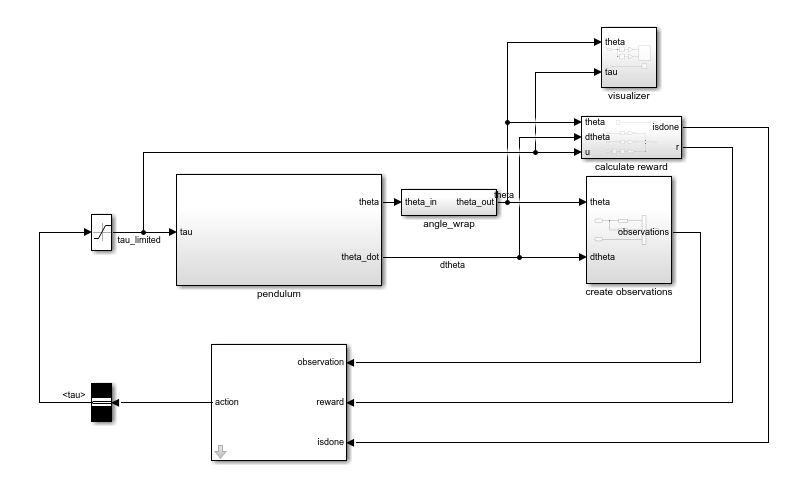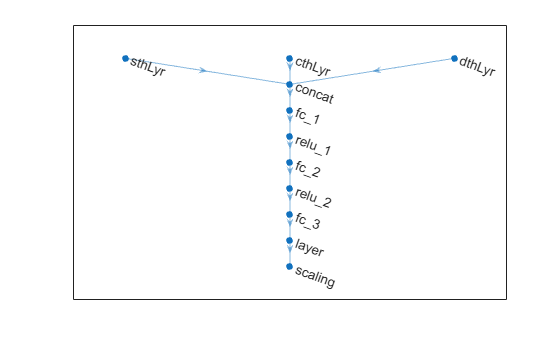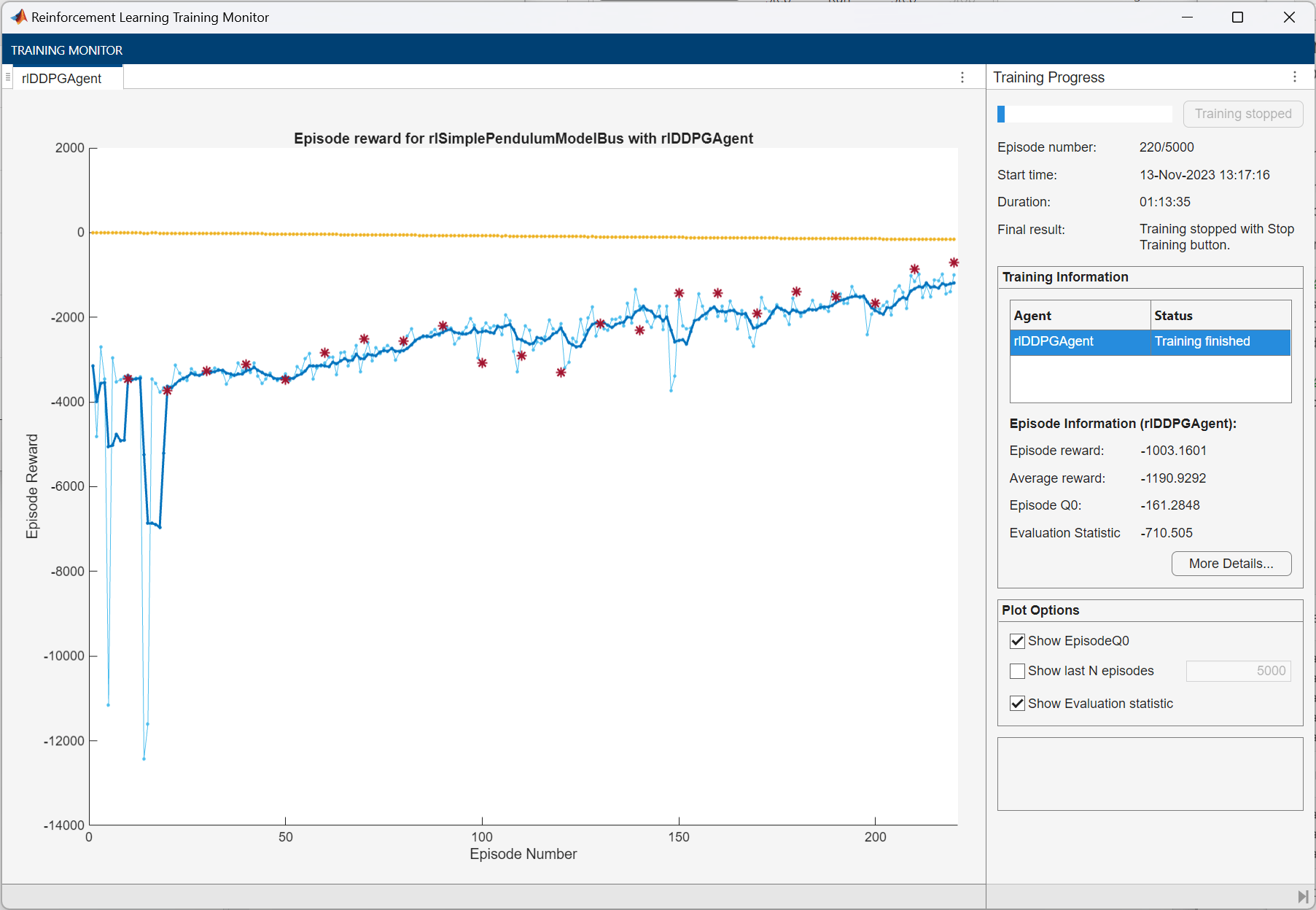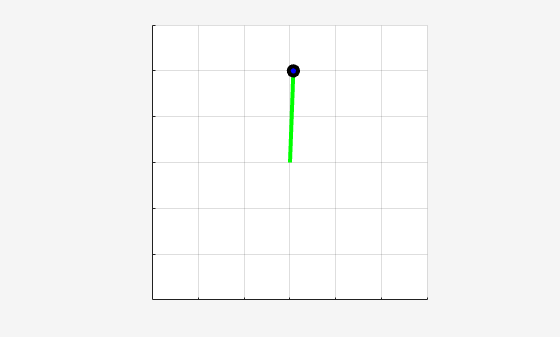# Train DDPG Agent to Swing Up and Balance Pendulum with Bus Signal

This example shows how to convert a simple frictionless pendulum Simulink® model to a reinforcement learning environment interface, and how to train a deep deterministic policy gradient (DDPG) agent in this environment.

For more information on DDPG agents, see Deep Deterministic Policy Gradient (DDPG) Agents. For an example showing how to train a DDPG agent in MATLAB®, see Compare DDPG Agent to LQR Controller.

### Pendulum Swing-Up Model with Bus

The starting model for this example is a simple frictionless pendulum. The training goal is to make the pendulum stand upright without falling over using minimal control effort.

Open the model.

```mdl = "rlSimplePendulumModelBus"; open_system(mdl)```For this model:

• The upward balanced pendulum position is 0 radians, and the downward hanging position is `pi` radians.

• The torque action signal from the agent to the environment is from –2 to 2 N·m.

• The observations from the environment are the sine of the pendulum angle, the cosine of the pendulum angle, and the pendulum angle derivative.

• Both the observation and action signals are Simulink buses.

• The reward ${\mathit{r}}_{\mathit{t}}$, provided at every time step, is

`${\mathit{r}}_{\mathit{t}}=-\left({{\theta }_{\mathit{t}}}^{2}+0.1{\stackrel{˙}{{\theta }_{\mathit{t}}}}^{2}+0.001{\mathit{u}}_{\mathit{t}-1}^{2}\right)$`

Here:

• ${\theta }_{\mathit{t}}$ is the angle of displacement from the upright position.

• $\stackrel{˙}{{\theta }_{\mathit{t}}}$ is the derivative of the displacement angle.

• ${\mathit{u}}_{\mathit{t}-1}$ is the control effort from the previous time step.

The model used in this example is similar to the simple pendulum model described in Load Predefined Control System Environments. The difference is that the model in this example uses Simulink buses for the action and observation signals.

### Create Environment Interface with Bus

The environment interface from a Simulink model is created using `rlSimulinkEnv`, which requires the name of the Simulink model, the path to the agent block, and observation and action reinforcement learning data specifications. For models that use bus signals for actions or observations, you can create the corresponding specifications using the `bus2RLSpec` function.

Specify the path to the agent block.

`agentBlk = "rlSimplePendulumModelBus/RL Agent";`

Create the observation `Bus` object. The channel names must correspond to the signal names specified in the Simulink buses used to create the observation and action signals.

```obsBus = Simulink.Bus(); obs(1) = Simulink.BusElement; obs(1).Name = "sin_theta"; obs(2) = Simulink.BusElement; obs(2).Name = "cos_theta"; obs(3) = Simulink.BusElement; obs(3).Name = "dtheta"; obsBus.Elements = obs;```

Create the action `Bus` object.

```actBus = Simulink.Bus(); act(1) = Simulink.BusElement; act(1).Name = "tau"; act(1).Min = -2; act(1).Max = 2; actBus.Elements = act;```

Create the action and observation specification objects using the Simulink buses.

`obsInfo = bus2RLSpec("obsBus","Model",mdl)`
```obsInfo=1×3 object 1x3 rlNumericSpec array with properties: LowerLimit UpperLimit Name Description Dimension DataType ```
`actInfo = bus2RLSpec("actBus","Model",mdl)`
```actInfo = rlNumericSpec with properties: LowerLimit: -2 UpperLimit: 2 Name: "tau" Description: "" Dimension: [1 1] DataType: "double" ```

Create the reinforcement learning environment for the pendulum model.

`env = rlSimulinkEnv(mdl,agentBlk,obsInfo,actInfo);`

To define the initial condition of the pendulum as hanging downward, specify an environment reset function using an anonymous function handle. This reset function sets the model workspace variable `theta0` to `pi`.

`env.ResetFcn = @(in)setVariable(in,"theta0",pi,"Workspace",mdl);`

Specify the simulation time `Tf` and the agent sample time `Ts` in seconds.

```Ts = 0.05; Tf = 20;```

Fix the random generator seed for reproducibility.

`rng(0)`

### Create DDPG Agent

DDPG agents use a parametrized deterministic policy over continuous action spaces, which is learned by a continuous deterministic actor. This actor takes the current observation as input and returns as output an action that is a deterministic function of the observation.

To model the parametrized policy within the actor, use a neural network with three input layers (each receiving one scalar observation, as specified by `obsInfo`) and one output layer (which returns the action to the environment action channel, as specified by `actInfo`).

Define the network as an array of layer objects, and combine the outputs from the input layes using a `concatenationLayer`. Since the output of `tanhLayer` is limited between -1 and 1, scale the network output to the range of the action using `scalingLayer`.

For more information on creating actors based on deep neural networks, see Create Policies and Value Functions.

```% Define input layers sinThetaInputLayer = featureInputLayer(1,Name="sin_th_lyr"); cosThetaInputLayer = featureInputLayer(1,Name="cos_th_lyr"); dThetaInputLayer = featureInputLayer(1,Name="dth_lyr"); % Define common path commonPath = [ concatenationLayer(1,3,Name="concat") fullyConnectedLayer(400) reluLayer fullyConnectedLayer(300) reluLayer fullyConnectedLayer(1) tanhLayer scalingLayer(Scale=max(actInfo.UpperLimit))]; % Create layergraph object and add layers actorNetwork = layerGraph(sinThetaInputLayer); actorNetwork = addLayers(actorNetwork,cosThetaInputLayer); actorNetwork = addLayers(actorNetwork,dThetaInputLayer); actorNetwork = addLayers(actorNetwork,commonPath); % Connect layers actorNetwork = connectLayers(actorNetwork,"sin_th_lyr","concat/in1"); actorNetwork = connectLayers(actorNetwork,"cos_th_lyr","concat/in2"); actorNetwork = connectLayers(actorNetwork,"dth_lyr","concat/in3");```

Convert to a `dlnetwork` object and display the number of weights.

```actorNetwork = dlnetwork(actorNetwork); summary(actorNetwork)```
``` Initialized: true Number of learnables: 122.2k Inputs: 1 'sin_th_lyr' 1 features 2 'cos_th_lyr' 1 features 3 'dth_lyr' 1 features ```

View the actor network configuration.

```figure plot(layerGraph(actorNetwork))```Create the actor using the specified deep neural network. Specify the action and observation info for the actor, as well as the names of the network input layers to be connected with the observation channels. For more information, see `rlContinuousDeterministicActor`.

```actor = rlContinuousDeterministicActor(actorNetwork,obsInfo,actInfo,... "ObservationInputNames",["sin_th_lyr","cos_th_lyr","dth_lyr"]);```

DDPG agents use a parametrized Q-value function approximator to estimate the value of the policy. A Q-value function critic takes the current observation and an action as inputs and returns a single scalar as output (the estimated discounted cumulative long-term reward for which receives the action from the state corresponding to the current observation, and following the policy thereafter).

To model the parametrized Q-value function within the critic, use a neural network with four input layers (three for the observation channels, as specified by `obsInfo`, and the other for the action channel, as specified by `actInfo`) and one output layer (which returns the scalar value).

Define each network path as an array of layer objects, assigning names to the input and output layers of each path. Use the input layers previously defined for the actor.

```% Observation path obsPath = [ concatenationLayer(1,3,Name="concat") fullyConnectedLayer(400) reluLayer fullyConnectedLayer(300,Name="obsPathOutLyr") ]; % Action path actPath = [ featureInputLayer(1,Name= "action") fullyConnectedLayer(300, ... Name="actPathOutLyr", ... BiasLearnRateFactor=0) ]; % Common path commonPath = [ additionLayer(2,Name="add") reluLayer fullyConnectedLayer(1,Name="CriticOutput") ]; % Create layergraph object and add layers criticNetwork = layerGraph(sinThetaInputLayer); criticNetwork = addLayers(criticNetwork,cosThetaInputLayer); criticNetwork = addLayers(criticNetwork,dThetaInputLayer); criticNetwork = addLayers(criticNetwork,actPath); criticNetwork = addLayers(criticNetwork,obsPath); criticNetwork = addLayers(criticNetwork,commonPath); % Connect Layers criticNetwork = connectLayers(criticNetwork,"sin_th_lyr","concat/in1"); criticNetwork = connectLayers(criticNetwork,"cos_th_lyr","concat/in2"); criticNetwork = connectLayers(criticNetwork,"dth_lyr","concat/in3"); criticNetwork = connectLayers(criticNetwork,"obsPathOutLyr","add/in1"); criticNetwork = connectLayers(criticNetwork,"actPathOutLyr","add/in2");```

Convert to `dlnetwork` and display the number of weights.

```net = dlnetwork(criticNetwork); %summary(criticNetwork)```

Create the critic approximator object using `criticNet`, the environment observation and action specifications, and the names of the network input layers to be connected with the environment observation and action channels. For more information, see `rlQValueFunction`.

```critic = rlQValueFunction(criticNetwork, ... obsInfo,actInfo,... ObservationInputNames=["sin_th_lyr","cos_th_lyr","dth_lyr"], ... ActionInputNames="action");```

Specify options for the critic representation using `rlOptimizerOptions`.

```actorOptions = rlOptimizerOptions(LearnRate=1e-4,GradientThreshold=1); criticOptions = rlOptimizerOptions(LearnRate=1e-03,GradientThreshold=1);```

To create the DDPG agent, first specify the DDPG agent options using `rlDDPGAgentOptions`.

```agentOpts = rlDDPGAgentOptions(... SampleTime=Ts,... ActorOptimizerOptions=actorOptions,... CriticOptimizerOptions=criticOptions,... ExperienceBufferLength=1e6,... MiniBatchSize=128); agentOpts.NoiseOptions.Variance = 0.6; agentOpts.NoiseOptions.VarianceDecayRate = 1e-5;```

Then create the DDPG agent using the specified actor representation, critic representation, and agent options. For more information, see `rlDDPGAgent`.

`agent = rlDDPGAgent(actor,critic,agentOpts);`

### Train Agent

To train the agent, first specify the training options. For this example, use the following options:

• Run each training for at most 50000 episodes, with each episode lasting at most `ceil(Tf/Ts)` time steps.

• Display the training progress in the Episode Manager dialog box (set the `Plots` option) and disable the command line display (set the `Verbose` option to `false`).

• Stop training when the agent receives an average cumulative reward greater than –740 over five consecutive episodes. At this point, the agent can quickly balance the pendulum in the upright position using minimal control effort.

• Save a copy of the agent for each episode where the cumulative reward is greater than –740.

For more information, see `rlTrainingOptions`.

```maxepisodes = 5000; maxsteps = ceil(Tf/Ts); trainOpts = rlTrainingOptions(... MaxEpisodes=maxepisodes,... MaxStepsPerEpisode=maxsteps,... ScoreAveragingWindowLength=5,... Verbose=false,... Plots="training-progress",... StopTrainingCriteria="AverageReward",... StopTrainingValue=-740);```

Train the agent using the `train` function. Training this agent is a computationally intensive process that takes several hours to complete. To save time while running this example, load a pretrained agent by setting `doTraining` to `false`. To train the agent yourself, set `doTraining` to `true`.

```doTraining = false; if doTraining % Train the agent. trainingStats = train(agent,env,trainOpts); else % Load the pretrained agent for the example. load("SimulinkPendBusDDPG.mat","agent") end```### Simulate DDPG Agent

To validate the performance of the trained agent, simulate it within the pendulum environment. For more information on agent simulation, see `rlSimulationOptions` and `sim`.

```simOptions = rlSimulationOptions(MaxSteps=500); experience = sim(env,agent,simOptions);```